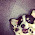Index

 STA & SI Chapter1 Chapter2 Chapter3 Chapter4 Chapter5 Chapter6 Chapter7 Chapter8 Introduction Static Timing Analysis Clock Advance STA Signal Integrity EDA Tools Timing Models Other Topics

 Extraction & DFM Chapter1 Chapter2 Chapter3 Chapter4 Chapter5 Chapter6 Introduction Parasitic Interconnect Corner (RC Corner) Manufacturing Effects and Their Modeling Dielectric Layer Process Variation Other Topic

Monday, January 1, 2018

AOI (AND-OR-INVERTER) Cell

AOI also known as AND-OR-Inverter.

AND-OR-Invert (AOI) logic or say gates are two-level logic functions constructed from the combination of one or more AND gates followed by a NOR gate. If we construct AND, OR and NOT gate separately, Number of transistor in AOI gates are less.

You might be thinking why need individual logic gate, why can't we implement it using just 2 AND gate and 1 NOR gate. Yes, you are right. But now think from CMOS point of view. In CMOS, we can implement AND gate using 1 CMOS NAND gate and 1 Inverter. It means above 2 AND gate changes into 2 NAND and 2 Inverter. I hope this explanation rang a bell in your mind. If not, keep patience (this is only I am going to explain in this article :) ).
Let's take an example and try to understand it.

For example: 2-2 AOI gate: ((A.B) + (C.D))'

Let's see how this function be implemented using logic gates (separately) Vs AOI cells.

Using Individual NAND, NOT and NOR Gate:
First we have to change the function as per logic gates availability. Y== ((A.B) + (C.D))' == (((A.B)')' + ((C.D)')')'

Function (((A.B)')' + ((C.D)')')' can be implemented as:
• NAND Gates:
• 2 NAND gates: 1st for (A.B)' and 2nd for (C.D)' (Assume X=(A.B)' and Y=(C.D)')
• 1 NAND gate uses 2 PMOS transistor and 2 NMOS transistor.
• So, total Transistors in 2 2-input NAND gate are 8 Transistors.
• Inverter:
• 2 Inverter: 1st (X)' and 2nd for (Y)'
• 1 Inverter uses 1 PMOS and 1 NMOS
• So, total Transistors in 1 Inverter are 2 Transistors.
• NOR Gates:
• 1 NOR Gate: (X' + Y')'
• 1 NOR gate uses 2 PMOS transistor and 2 NMOS transistor.
• So, total Transistors in 1 2-input NOR gate are 4 Transistors.

Total Transistor in case of Individually implementing ((A.B) + (C.D))' = 14 Transistor.

CMOS Representation of above function is given below.

Using AOI logic gates.
Implementation using AOI cells are very easy. In this case, we are not suppose to change the function. Y == ((A.B) + (C.D))'

Below diagram can help you to understand how cells can implement using CMOS. In this case, total Transistor require are 8 Transistor. If you are not able to understand this part (I am sure you need to refresh your concept for CMOS circuit).

From above explanation, I am sure you are now in position to understand the importance of AOI cells. These cells are so important that in Standard cell library, you can easily find these cells (as other logic gates). So in short, I can say that these are also part of STANDARD Cells (So don't assume that only NAND, AND, OR, Buffer, Inverter, XOR, XNOR are Standard cells).

In a similar fashion, you can take any example and try to understand how many transistors are required in case of implementing that function in AOI form. This type of questions are very common during Interview or Written test.

Less number of Transistors for implementing a particular logic function helps in multiple ways. Like
• Increased speed: Less transistor means less delay, means fast response time.
• Reduced Power: Less number of transistor means less power consumption.
• Smaller area: Less number of transistor means less area consumption.
• Potentially lower fabrication cost: Fabrication cost is also less because of less number of manufacturing of transistor.

You can also understand OAI cells in similar way OR if you are not able to .. then wait for my Article. :)

1 comment:

1.Wow! It's so complicated :o Thank you for sharing!
piknu